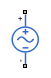# Sinusoidal Voltage Source

Damped sinusoidal voltage source

•Libraries:
Simscape / Electrical / Additional Components / SPICE Sources

## Description

The Sinusoidal Voltage Source block represents a damped sinusoidal voltage source whose output voltage is independent of the current through the source. The following equations describe the output as a function of time:

`$\begin{array}{l}{V}_{out}\left(Time\le TD\right)=VO\\ {V}_{out}\left(Time>TD\right)=VO+VA*{e}^{-\left(Time-TD\right)*DF}*\mathrm{sin}\left(2\pi *FREQ*\left(Time-TD\right)\right)\end{array}$`

where:

• V0 is the Voltage offset, VO parameter value.

• VA is the Sinusoidal amplitude, VA parameter value.

• FREQ is the Sinusoidal frequency, FREQ parameter value.

• TD is the Time delay, TD parameter value.

• DF is the Damping factor, DF parameter value.

## Ports

### Conserving

expand all

Electrical conserving port associated with the sinusoidal voltage source positive voltage

Electrical conserving port associated with the sinusoidal voltage source negative voltage

## Parameters

expand all

Magnitude of the time-independent part of the output voltage.

Magnitude of the sinusoidal part of the output voltage.

Frequency of the output sine wave. The value can be less than `0`.

Time at which the sine wave first starts. A value less than `0` indicates a phase shift of 180 degrees.

Exponential damping factor for the sine wave to produce the output voltage. If DF is greater than or equal to `0`, the exponential part of the equation is always damping for time greater than TD. The value must be greater than or equal to `0`.

## Version History

Introduced in R2008a